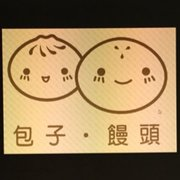{meta: 原调: 1=C} {meta: 节拍: 2/4} {comment: 间奏} [C] |[D] |[Am] |[D] | [F] |[G] |[Em] [D] |[C] |[C] {comment: 主歌 A} [C]牵你的[D]手 [Am]放在我[D]手 [F]我的心[C]里 [F]只有一个[G]你 [C]温你的[D]脸 [Am]吻你的[D]唇 [F]想要和你[C]一起[F]每分每[G]秒 {comment: 副歌} [F]问老天为[G]什么我们[Em]彼此[D]会相[C]遇 [F]问我们心里都[G]多想在[C]一起 {comment: 间奏} [C] |[D] |[Am] |[D] | [F] |[G] |[Em] [D] |[C] |[C] {comment: 扫弦} {comment: 主歌 A} [C]牵你的[D]手 [Am]放在我[D]手 [F]我的心[C]里 [F]只有一个[G]你 [C]温你的[D]脸 [Am]吻你的[D]唇 [F]想要和你[C]一起[F]每分每[G]秒 {comment: 副歌} [F]问老天为[G]什么我们[Em]彼此[D]会相[C]遇 [F]问我们心里都[G]多想在[C]一起 [F]问老天为[G]什么我们[Em]彼此[D]会相[C]遇 [F]问我们心里都[G]多想在[C]一起 {title:相遇} {artist:原创歌曲} {author:小包子}